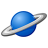# QlikView App Dev

Discussion Board for collaboration related to QlikView App Development.

Announcements
Join us at the Cloud Data and Analytics Tour! REGISTER TODAY
cancel
Showing results for
Search instead for
Did you mean:Creator III

## Set Analysis

Hi There

I need to sum a variable and use set analysis to specify the parameters to sum of that variable...

Is it possible to use Set analysis like below??? or something like it... when doing it the way I have I just get the full sum of the variable it is disregarding the set analysis which I have specified.

vGrossProfit= Sum(Sales)-Sum([Cost of Sales])

vShop1GrossProfit=Sum({<Division={'Shop1'}>}vGrossProfit)

Sum({<Desk={'Desk1'}>}vShop1GrossProfit)

Thank You

1 Solution

Accepted SolutionsMVP & Luminary

I don't understand how your approach could simplify (main reason for using variables) anything. Would it not easier to use something like this:

Sum({<Division={'Shop1'}, Desk={'Desk1'}>} Sales)-Sum({<Division={'Shop1'}, Desk={'Desk1'}>} [Cost of Sales])

or

var:

Sum({<Division={'Shop1'}, Desk={'Desk1'}>} \$1)

and then

\$(var(Sales)) - \$(var([Cost of Sales]))

- Marcus

3 RepliesMVP

Unfortunately, the 2nd and 3rd expressions are not going to work like thisCreator III
Author

Would I have to do the set analysis for each scenario 1st and then create the variables?MVP & Luminary

I don't understand how your approach could simplify (main reason for using variables) anything. Would it not easier to use something like this:

Sum({<Division={'Shop1'}, Desk={'Desk1'}>} Sales)-Sum({<Division={'Shop1'}, Desk={'Desk1'}>} [Cost of Sales])

or

var:

Sum({<Division={'Shop1'}, Desk={'Desk1'}>} \$1)

and then

\$(var(Sales)) - \$(var([Cost of Sales]))

- Marcus